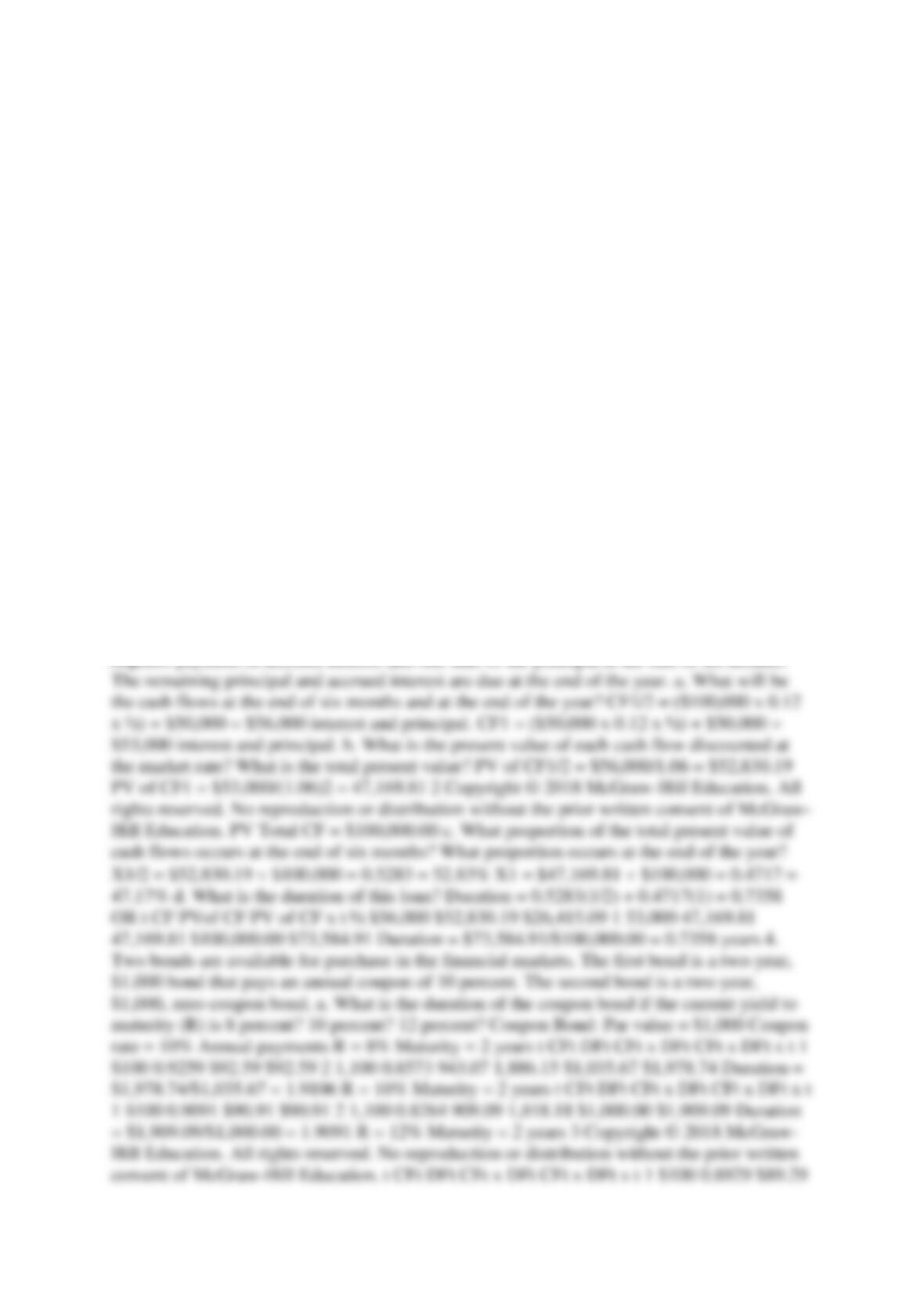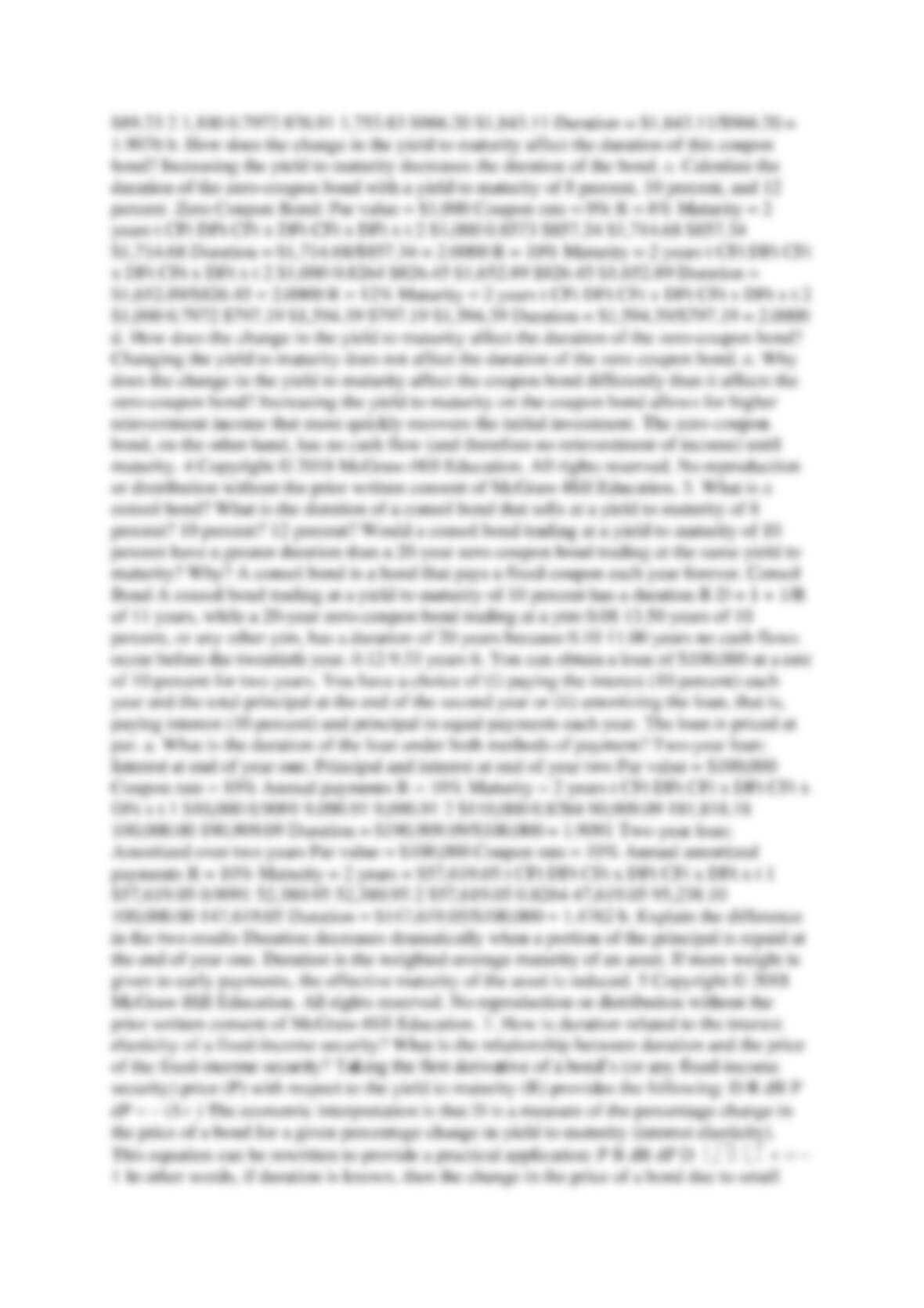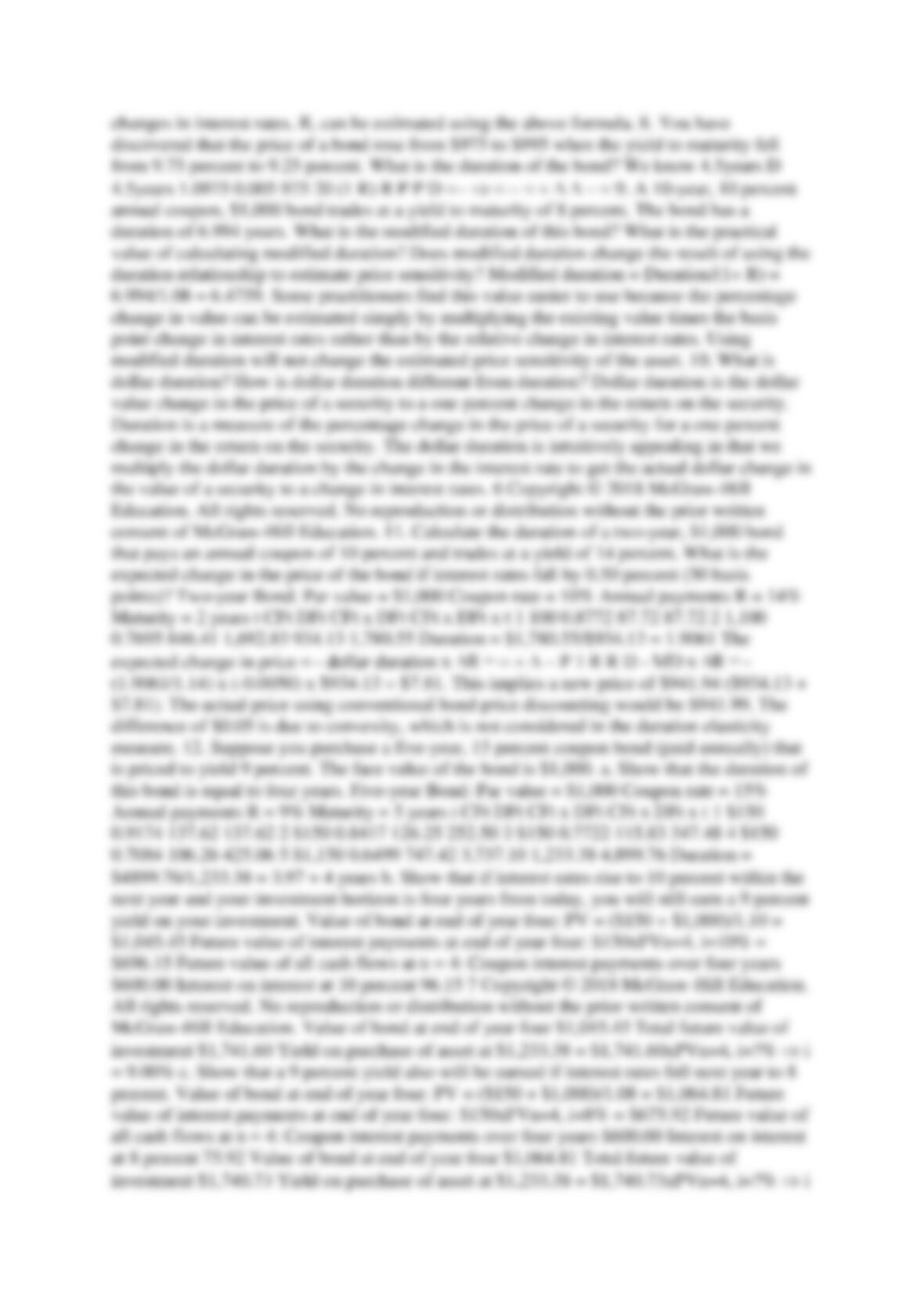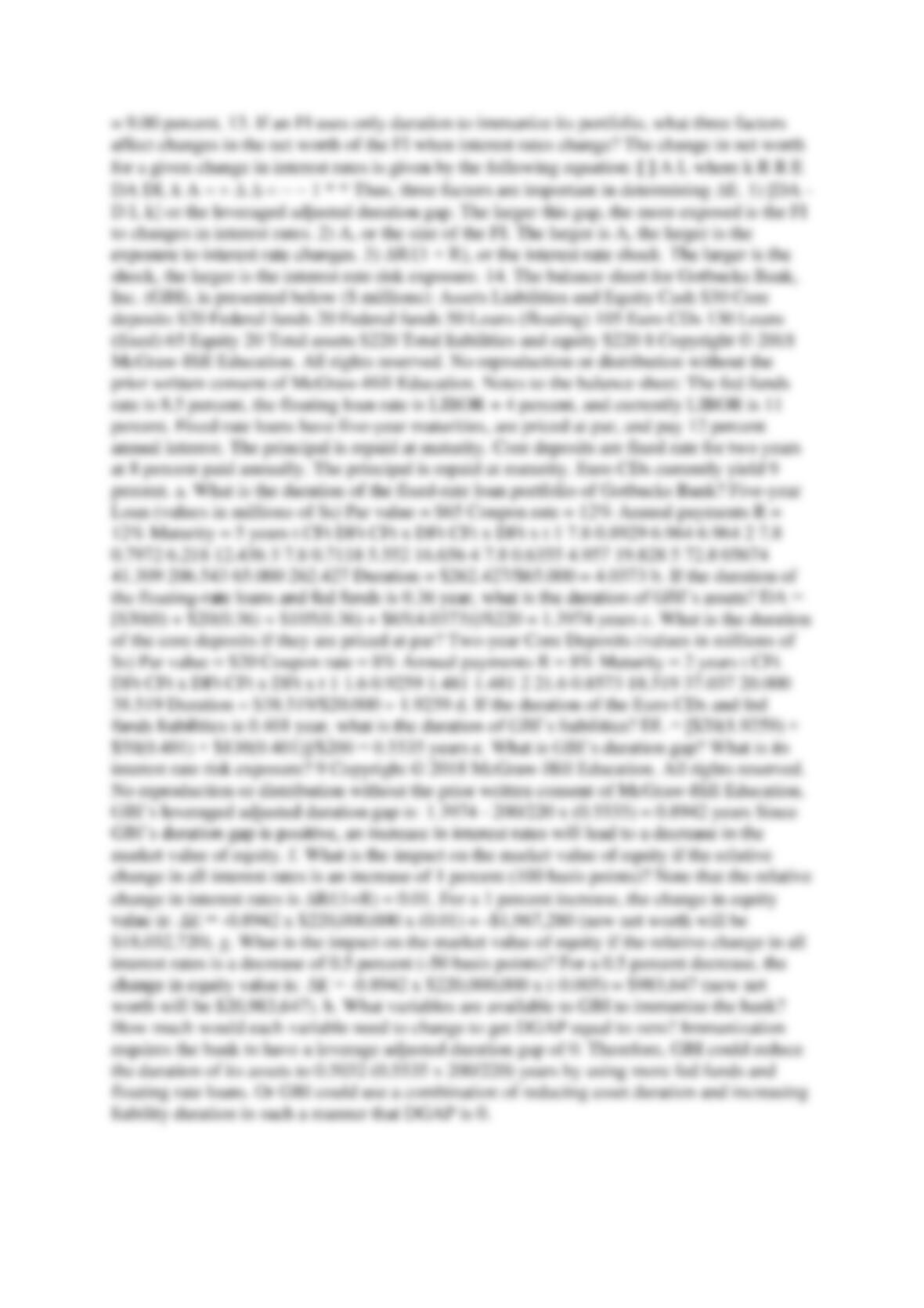finance

Type
Essay
Pages
4
Word Count
2586
School
waikato univeristy
Course
fiannce302Chapter Nine: Questions and Answers 1. What is the difference between book value
accounting and market value accounting? How do interest rate changes affect the value of
bank assets and liabilities under the two methods? What is marking to market? Book value
accounting reports assets and liabilities at the original issue values. Market value accounting
reports assets and liabilities at their current market values. Current market values may be
different from book values because they reflect current market conditions, such as current
interest rates. FIs generally report their balance sheets using book value accounting methods.
This is a problem if an asset or liability has to be liquidated immediately. If the asset or
liability is held until maturity, then the reporting of book values does not pose a problem. For
an FI, a major factor affecting asset and liability values is interest rate changes. If interest
rates increase, the value of both loans (assets) and deposits and other debt (liabilities) fall. If
assets and liabilities are held until maturity, interest rate changes do not affect the valuation of
the FI. However, if deposits or loans have to be refinanced, then market value accounting
presents a better picture of the condition of the FI. The process by which changes in the
economic value of assets and liabilities are accounted is called marking to market. The
changes can be beneficial as well as detrimental to the total economic value of the FI. 2. What
are the two different general interpretations of the concept of duration, and what is the
technical definition of this term? How does duration differ from maturity? Duration measures
the weighted-average life of an asset or liability in economic terms. As such, duration has
economic meaning as the interest rate sensitivity (or interest elasticity) of an asset’s value to
changes in the interest rate. Duration differs from maturity as a measure of interest rate
sensitivity because duration takes into account the time of arrival and the rate of reinvestment
of all cash flows during the assets life. Technically, duration is the weighted-average time to
maturity using the relative present values of the cash flows as the weights. 3. A one-year,
\$100,000 loan carries a coupon rate and a market interest rate of 12 percent. The loan
requires payment of accrued interest and one-half of the principal at the end of six months.
The remaining principal and accrued interest are due at the end of the year. a. What will be